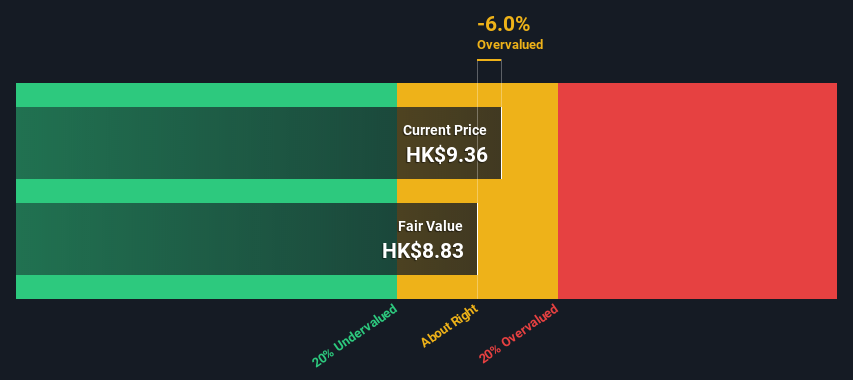Today we will run through one way of estimating the intrinsic value of Legend Holdings Corporation (HKG:3396) by taking the forecast future cash flows of the company and discounting them back to today’s value. This will be done using the Discounted Cash Flow (DCF) model. Models like these may appear beyond the comprehension of a lay person, but they’re fairly easy to follow.

We generally believe that a company’s value is the present value of all of the cash it will generate in the future. However, a DCF is just one valuation metric among many, and it is not without flaws. Anyone interested in learning a bit more about intrinsic value should have a read of the Simply Wall St analysis model.

View our latest analysis for Legend Holdings

### What’s the estimated valuation?

We’re using the 2-stage growth model, which simply means we take in account two stages of company’s growth. In the initial period the company may have a higher growth rate and the second stage is usually assumed to have a stable growth rate. To begin with, we have to get estimates of the next ten years of cash flows. Seeing as no analyst estimates of free cash flow are available to us, we have extrapolate the previous free cash flow (FCF) from the company’s last reported value. We assume companies with shrinking free cash flow will slow their rate of shrinkage, and that companies with growing free cash flow will see their growth rate slow, over this period. We do this to reflect that growth tends to slow more in the early years than it does in later years.

A DCF is all about the idea that a dollar in the future is less valuable than a dollar today, and so the sum of these future cash flows is then discounted to today’s value:

#### 10-year free cash flow (FCF) estimate

 2022 2023 2024 2025 2026 2027 2028 2029 2030 2031 Levered FCF (CN¥, Millions) CN¥5.11b CN¥3.00b CN¥2.15b CN¥1.73b CN¥1.50b CN¥1.37b CN¥1.29b CN¥1.25b CN¥1.22b CN¥1.21b Growth Rate Estimate Source Est @ -59.55% Est @ -41.24% Est @ -28.42% Est @ -19.45% Est @ -13.17% Est @ -8.78% Est @ -5.7% Est @ -3.55% Est @ -2.04% Est @ -0.98% Present Value (CN¥, Millions) Discounted @ 11% CN¥4.6k CN¥2.4k CN¥1.6k CN¥1.1k CN¥877 CN¥718 CN¥608 CN¥527 CN¥464 CN¥412

(“Est” = FCF growth rate estimated by Simply Wall St)
Present Value of 10-year Cash Flow (PVCF) = CN¥13b

After calculating the present value of future cash flows in the initial 10-year period, we need to calculate the Terminal Value, which accounts for all future cash flows beyond the first stage. For a number of reasons a very conservative growth rate is used that cannot exceed that of a country’s GDP growth. In this case we have used the 5-year average of the 10-year government bond yield (1.5%) to estimate future growth. In the same way as with the 10-year ‘growth’ period, we discount future cash flows to today’s value, using a cost of equity of 11%.

Terminal Value (TV)= FCF2031 × (1 + g) ÷ (r – g) = CN¥1.2b× (1 + 1.5%) ÷ (11%– 1.5%) = CN¥12b

Present Value of Terminal Value (PVTV)= TV / (1 + r)10= CN¥12b÷ ( 1 + 11%)10= CN¥4.2b

The total value is the sum of cash flows for the next ten years plus the discounted terminal value, which results in the Total Equity Value, which in this case is CN¥18b. In the final step we divide the equity value by the number of shares outstanding. Compared to the current share price of HK\$9.4, the company appears around fair value at the time of writing. The assumptions in any calculation have a big impact on the valuation, so it is better to view this as a rough estimate, not precise down to the last cent.SEHK:3396 Discounted Cash Flow May 3rd 2022

### The assumptions

Now the most important inputs to a discounted cash flow are the discount rate, and of course, the actual cash flows. Part of investing is coming up with your own evaluation of a company’s future performance, so try the calculation yourself and check your own assumptions. The DCF also does not consider the possible cyclicality of an industry, or a company’s future capital requirements, so it does not give a full picture of a company’s potential performance. Given that we are looking at Legend Holdings as potential shareholders, the cost of equity is used as the discount rate, rather than the cost of capital (or weighted average cost of capital, WACC) which accounts for debt. In this calculation we’ve used 11%, which is based on a levered beta of 2.000. Beta is a measure of a stock’s volatility, compared to the market as a whole. We get our beta from the industry average beta of globally comparable companies, with an imposed limit between 0.8 and 2.0, which is a reasonable range for a stable business.